#Model Fit audit

## Half-normal plot

The half-normal plot presented in this chapter is one of the tools designed to evaluate the goodness of fit of a statistical model. It is a graphical method for comparing two probability distributions by plotting their quantiles against each other.

Points on the plot correspond to ordered absolute values of model diagnostic (i.e. standardized residuals) plotted against theoretical order statistics from a half-normal distribution.

There are various implementations of half-normal plots in R. Functions for generating such plotes are available in packages auditor, faraway, hnp, but also in others. Some functions can only draw a simple half-normal plot, while some have additional functionalities like a simulated envelope and score of goodness-of-fit.

## auditor::plotHalfNormal()

library(auditor)
set.seed(123)


Function plotHalfNormal() offers a plotting interface for half-normal plots generated by hnp package in a unified style using ggplot2. Additional functionalities not included in the hnp are scores and the possibility to draw half-normal plot on a quantile scale.

Below we present example of the use of half-normal plots for a generalized linear models. Plots are generated by plotHalfNormal() function from package auditor.

By default, deviance residuals were used as diagnostic values.

# Use-case

We will use dataset corn from the hnp package. For more details on the data set and models see Moral, R., Hinde, J., & Demétrio, C. (2017). Half-Normal Plots and Overdispersed Models in R: The hnp Package.

data("corn", package = "hnp")


If diagnostic values are from the normal distribution, they are close to a straight line. However, if they don't come from a normal distribution, they still show a certain trend. Simulated envelopes can be used to help verify the correctness of this trend. For a well-fitted model, diagnostic values should lay within the envelope.

## Binomial model

First step of auditing is fitting a model and creating a modelAudit object which wraps up a model with meta-data.

model.bin <- glm(cbind(y, m - y) ~ extract, family = binomial, data = corn)

bin_au <- audit(model.bin, data = corn, y = corn$y)  modelAudit object may be plotted. plotHalfNormal(bin_au, sim=500)  ## Binomial model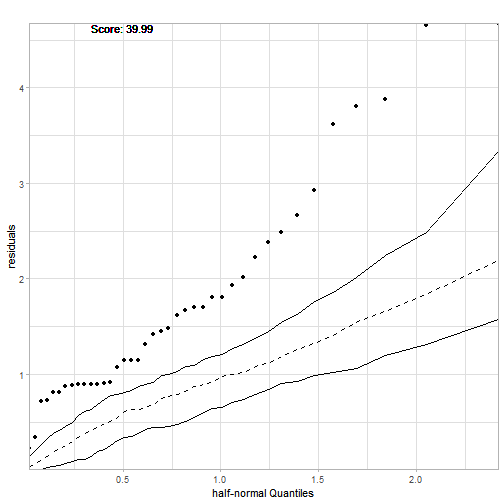It doesn't fit because of the overdispersion in data. An alternative way for generating plots is creating a modelFit object and then plotting it. This require additional line of code. However, once created modelFit contains all nessesary calculations that Half-Normal plot require. Among others simulated model residuals. model.bin <- glm(cbind(y, m - y) ~ extract, family = binomial, data = corn) bin_au <- audit(model.bin, data = corn, y = corn$y)

bin_mf <- modelFit(bin_au)

plot(bin_mf, sim=500)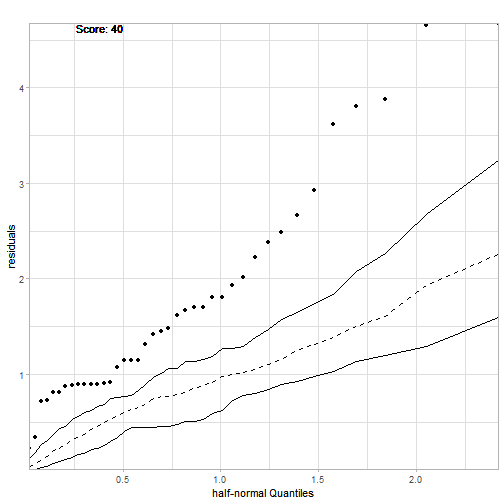## Other glm models

Next step is to try fitting overdispersion models: quasi-binomial, beta-binomial and logistic-normal.

### Quasi-binomial model

fit2_b <- glm(cbind(y, m - y) ~ extract, family = quasibinomial, data = corn)
fit2_b_au <- audit(fit2_b, data = corn, y = corn$y) plotHalfNormal(fit2_b_au, sim=500)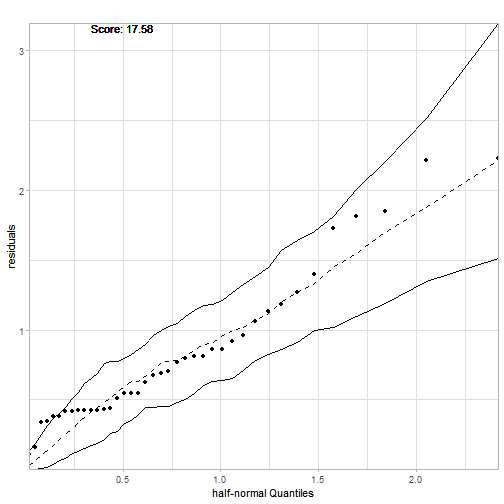### Beta-binomial model library(aods3) fit3_b <- aodml(cbind(y, m - y) ~ extract, family = "bb", data = corn) fit3_b_au <- audit(fit3_b, data = corn, y = corn$y)
plotHalfNormal(fit3_b_au, sim=500)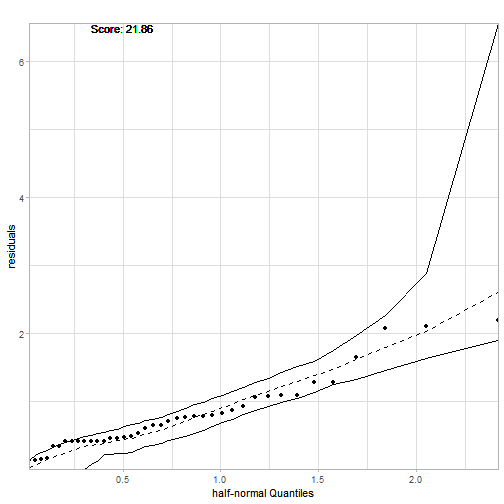### Logistic-normal model

x <- factor(seq_len(nrow(corn)))
fit4_b <- glmer(cbind(y, m - y) ~ extract + (1 | x), family = binomial, data = corn)
fit4_b_au <- audit(fit4_b, data = corn, y = corn$y) plotHalfNormal(fit4_b_au, sim=500)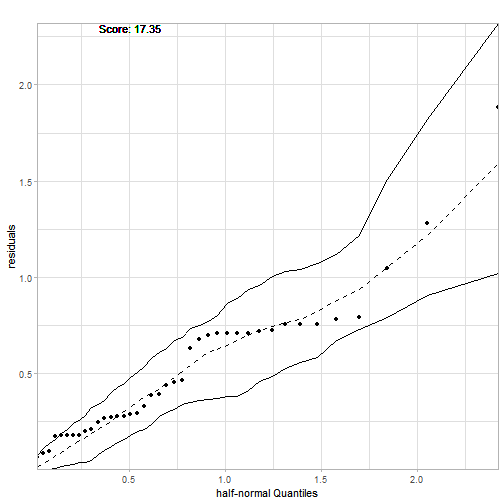The results of all models are quite similar. A useful tool to compare goodness-of-fit of two models is Score calculated by plotHalfNormal() function with parameter score==TRUE. Score is approximately:$ \sum{#[resi \leq simres{i,j}] - n }$with the distinction that each element of sum is also scaled to take values from $$[0,1]$$. $$res_i$$ is a residual for i-th observation, $$simres_{i,j}$$ is the residual of j-th simulation for i-th observation, and n is the number of simulations for each observation. Scores are calculated on the basis of simulated data, so they may differ between function calls. ## Quantile scale In function plotHalfNormal there is also a posibility to draw half-normal plot on a quantile scale. plotHalfNormal(fit2_b_au, quant.scale = TRUE)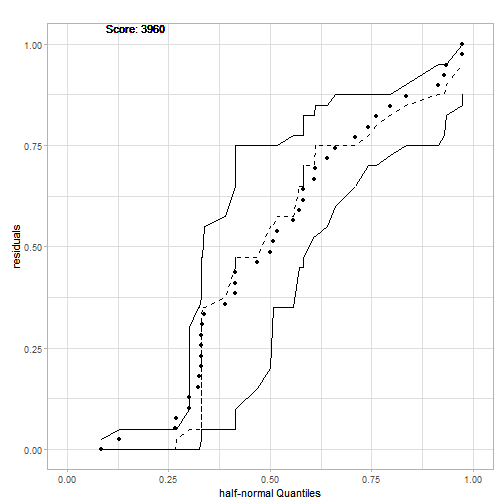# Classification HalfNormal plots works also for classification random Forest. In this case we consider the differences between observed class and predicted probabilities to be residuals. library(randomForest) iris_rf <- randomForest(Species ~ ., data=iris) iris_rf_au <- audit(iris_rf, data = iris, y = iris$Species)
plotHalfNormal(iris_rf_au)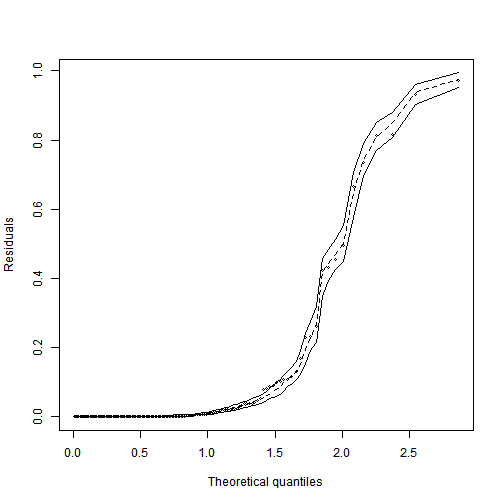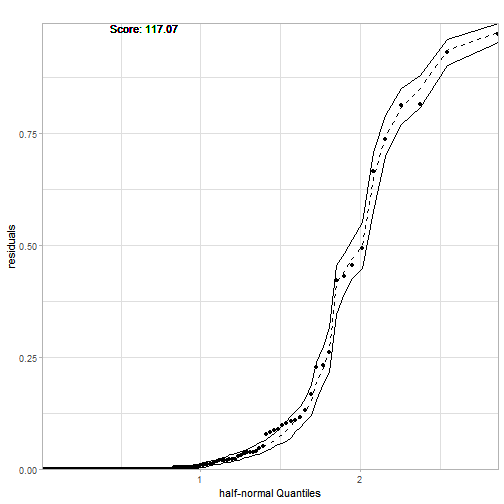# Other methods

Other methods and plots are described in vignettes:

# References

Moral, R., Hinde, J., & Demétrio, C. (2017). Half-Normal Plots and Overdispersed Models in R: The hnp Package. Journal of Statistical Software, 81(10), 1 - 23. doi:http://dx.doi.org/10.18637/jss.v081.i10